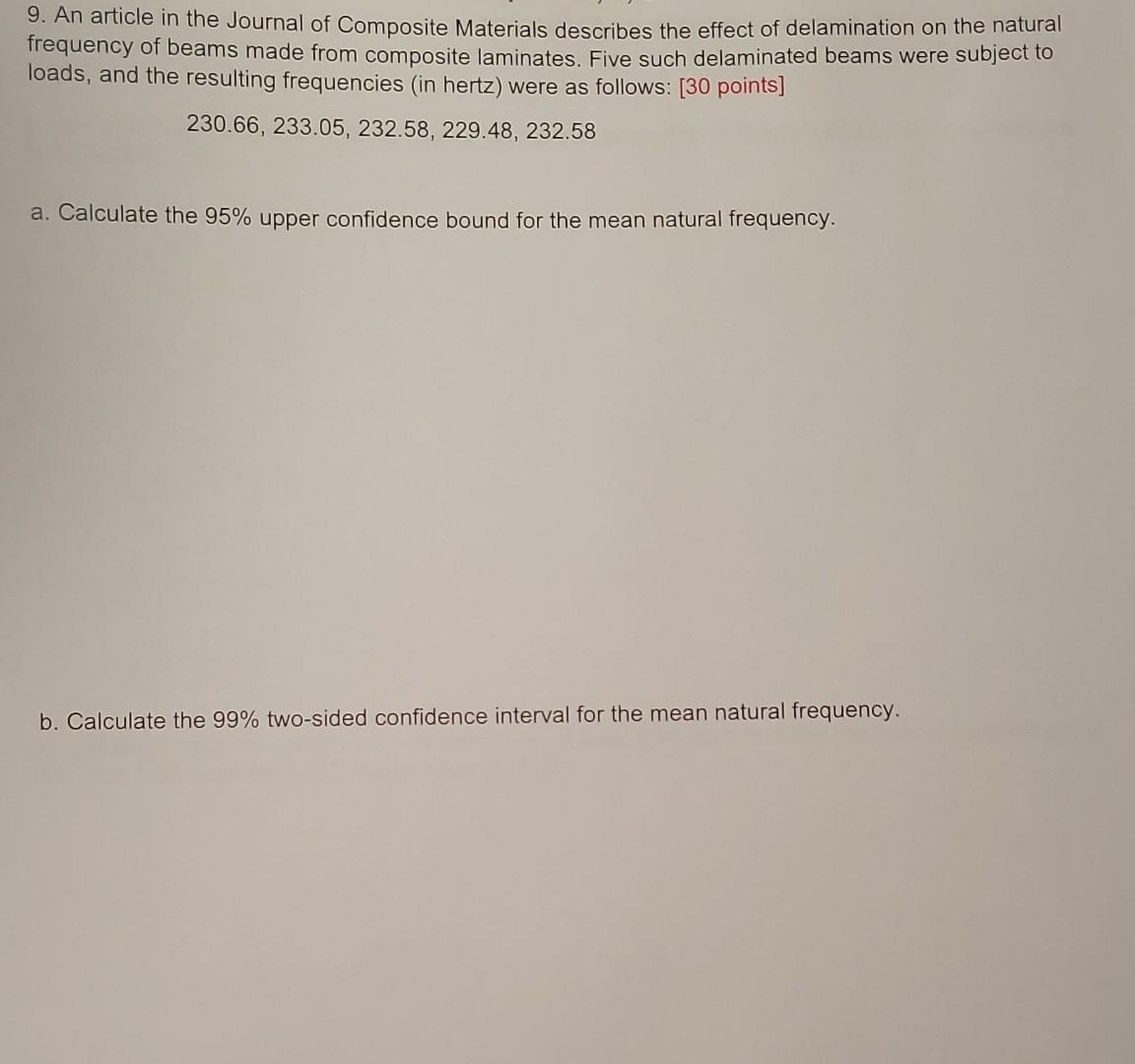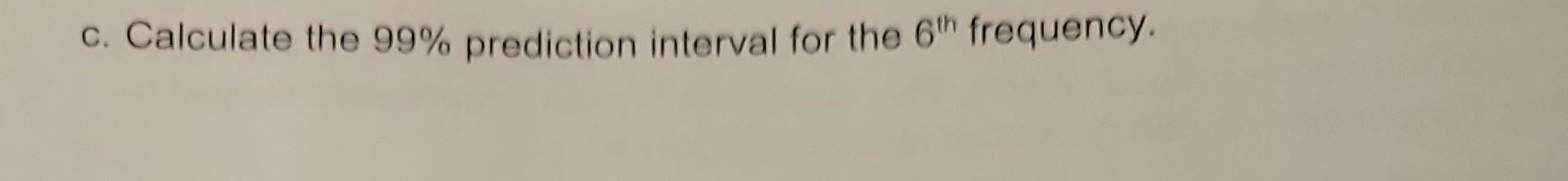Home / Expert Answers / Statistics and Probability / i-need-help-on-part-c-plz-9-an-article-in-the-journal-of-composite-materials-describes-th-pa228

# (Solved): I need help on Part C Plz 9. An article in the Journal of Composite Materials describes th ...I need help on Part C Plz

9. An article in the Journal of Composite Materials describes the effect of delamination on the natural frequency of beams made from composite laminates. Five such delaminated beams were subject to loads, and the resulting frequencies (in hertz) were as follows: [30 points] a. Calculate the upper confidence bound for the mean natural frequency. b. Calculate the two-sided confidence interval for the mean natural frequency. c. Calculate the prediction interval for the frequency.

We have an Answer from Expert